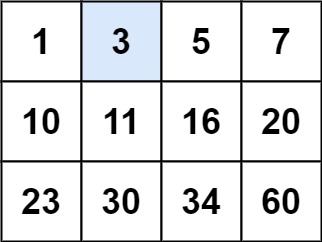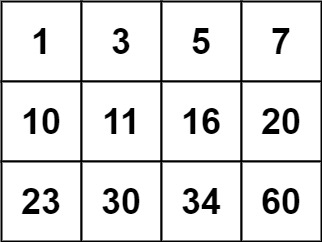# 74. Search a 2D Matrix / Medium

Write an efficient algorithm that searches for a value target in an m x n integer matrix matrix. This matrix has the following properties:

• Integers in each row are sorted from left to right.
• The first integer of each row is greater than the last integer of the previous row.

## Example 1:Input: matrix = [[1,3,5,7],[10,11,16,20],[23,30,34,60]], target = 3
Output: true

## Example 2:Input: matrix = [[1,3,5,7],[10,11,16,20],[23,30,34,60]], target = 13
Output: false

## Constraints:

• m == matrix.length
• n == matrix[i].length
• 1 <= m, n <= 100
• -10^4 <= matrix[i][j], target <= 10^4

# Solution: Binary Search

## 效能

### Complexity

• Time Complexity: O(log(mn))
• Space Complexity: O(1)

### LeetCode Result

• Runtime: 3 ms
• Memory Usage: 9.5 MB